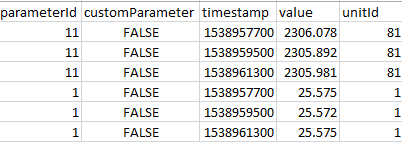# How to create a Pandas DataFrame from another DataFrame's column, which is a list

Hi, I have a python script that is creating a DataFrame from some json data.
I can create a DataFrame (df) from the data, but I need to create a DataFrame from the 'readings' column within the df DataFrame. My code is failing because the 'readings' column is a list.
Ultimately I need to create a DataFrame with the two DataFrames combined:This is the python code I'm working with:

``````import pandas as pd
import json

data = {
'locationId':123546987,
'parameters':[
{
'parameterId':'11',
'unitId':'81',
'customParameter':False,
'readings':[
{
'timestamp':1538957700,
'value':2306.078
},
{
'timestamp':1538959500,
'value':2305.892
},
{
'timestamp':1538961300,
'value':2305.981
}
]
},
{
'parameterId':'1',
'unitId':'1',
'customParameter':False,
'readings':[
{
'timestamp':1538957700,
'value':25.575
},
{
'timestamp':1538959500,
'value':25.572
},
{
'timestamp':1538961300,
'value':25.575
}
]
}
]
}

val = json.loads(json.dumps(data))

val1 = val['parameters']

#val2 = [{'timestamp': 1538957700, 'value': 2306.078}, {'timestamp': 1538959500, 'value': 2305.892}, {'timestamp': 1538961300, 'value': 2305.981}]
val2 = val1['readings']

df = pd.DataFrame(val1).set_index('parameterId')
print(df)

df2 = pd.DataFrame(val2)
print(df2)
``````

Thank you
Data Management SpecialistAsked:
###### Who is Participating?
I wear a lot of hats...

"The solutions and answers provided on Experts Exchange have been extremely helpful to me over the last few years. I wear a lot of hats - Developer, Database Administrator, Help Desk, etc., so I know a lot of things but not a lot about one thing. Experts Exchange gives me answers from people who do know a lot about one thing, in a easy to use platform." -Todd S.

Excel & VBA ExpertCommented:
I am not sure if this is the right way of doing this but you may try something like this...

``````import pandas as pd
import json

data = {
'locationId':123546987,
'parameters':[
{
'parameterId':'11',
'unitId':'81',
'customParameter':False,
'readings':[
{
'timestamp':1538957700,
'value':2306.078
},
{
'timestamp':1538959500,
'value':2305.892
},
{
'timestamp':1538961300,
'value':2305.981
}
]
},
{
'parameterId':'1',
'unitId':'1',
'customParameter':False,
'readings':[
{
'timestamp':1538957700,
'value':25.575
},
{
'timestamp':1538959500,
'value':25.572
},
{
'timestamp':1538961300,
'value':25.575
}
]
}
]
}

print(data)

val = json.loads(json.dumps(data))
val = pd.DataFrame(val)
print(val)

def getReadings(dict):
d = pd.DataFrame(dict['readings'])
d['unitId'] = dict['unitId']
d['parameterId'] = dict['parameterId']
return d

df = pd.DataFrame()
for i in range(len(val)):
df1 = getReadings(val.iloc[i,1])
df1['locationId'] = val.iloc[i,0]
df1['customParameter'] = 'False'
df = df.append(df1)

print(df)
``````
Analyst Assistant Commented:
Building on what Subodh posted, try this.
``````val = json.loads(json.dumps(data))
val = pd.DataFrame(val)

def getReadings(dict):
d = pd.DataFrame(dict['readings'], columns=['parameterId','customParameter','timestamp','value','unitId'])
d['parameterId'] = dict['parameterId']
d['customParameter'] = dict['customParameter']
d['unitId'] = dict['unitId']
return d

df = pd.DataFrame()

for i in range(len(val)):
df1 = getReadings(val.iloc[i,1])
df = df.append(df1)

print(df)
``````
Excel & VBA ExpertCommented:
I missed rearranging the columns as per the desired output. :)

Here is the revised code...

``````import pandas as pd
import json

data = {
'locationId':123546987,
'parameters':[
{
'parameterId':'11',
'unitId':'81',
'customParameter':False,
'readings':[
{
'timestamp':1538957700,
'value':2306.078
},
{
'timestamp':1538959500,
'value':2305.892
},
{
'timestamp':1538961300,
'value':2305.981
}
]
},
{
'parameterId':'1',
'unitId':'1',
'customParameter':False,
'readings':[
{
'timestamp':1538957700,
'value':25.575
},
{
'timestamp':1538959500,
'value':25.572
},
{
'timestamp':1538961300,
'value':25.575
}
]
}
]
}

print(data)

val = json.loads(json.dumps(data))
val = pd.DataFrame(val)
print(val)

def getReadings(dict):
d = pd.DataFrame(dict['readings'])
d['unitId'] = dict['unitId']
d['parameterId'] = dict['parameterId']
return d

df = pd.DataFrame()
for i in range(len(val)):
df1 = getReadings(val.iloc[i,1])
df1['locationId'] = val.iloc[i,0]
df1['customParameter'] = 'False'
df = df.append(df1)

df = df[['parameterId','customParameter','timestamp','value','unitId']]
print(df)
``````
Analyst Assistant Commented:
Subdoh

Why do you seem to be setting customParameter to 'False' for each row?

Shouldn't it's value come from the data?:)
Excel & VBA ExpertCommented:
@Norie

You are right. Didn't notice that column in the data. Thanks for pointing that out. :)

``````import pandas as pd
import json

data = {
'locationId':123546987,
'parameters':[
{
'parameterId':'11',
'unitId':'81',
'customParameter':False,
'readings':[
{
'timestamp':1538957700,
'value':2306.078
},
{
'timestamp':1538959500,
'value':2305.892
},
{
'timestamp':1538961300,
'value':2305.981
}
]
},
{
'parameterId':'1',
'unitId':'1',
'customParameter':False,
'readings':[
{
'timestamp':1538957700,
'value':25.575
},
{
'timestamp':1538959500,
'value':25.572
},
{
'timestamp':1538961300,
'value':25.575
}
]
}
]
}

val = json.loads(json.dumps(data))
val = pd.DataFrame(val)

def getReadings(dict):
d = pd.DataFrame(dict['readings'])
d['customParameter'] = dict['customParameter']
d['unitId'] = dict['unitId']
d['parameterId'] = dict['parameterId']
return d

df = pd.DataFrame()
for i in range(len(val)):
df1 = getReadings(val.iloc[i,1])
df1['locationId'] = val.iloc[i,0]
df = df.append(df1)

df = df[['parameterId','customParameter','timestamp','value','unitId']]
print(df)
``````

Experts Exchange Solution brought to you by

Your issues matter to us.

Facing a tech roadblock? Get the help and guidance you need from experienced professionals who care. Ask your question anytime, anywhere, with no hassle.

###### It's more than this solution.Get answers and train to solve all your tech problems - anytime, anywhere.Try it for free Edge Out The Competitionfor your dream job with proven skills and certifications.Get started today Stand Outas the employee with proven skills.Start learning today for free Move Your Career Forwardwith certification training in the latest technologies.Start your trial today
python3

From novice to tech pro — start learning today.